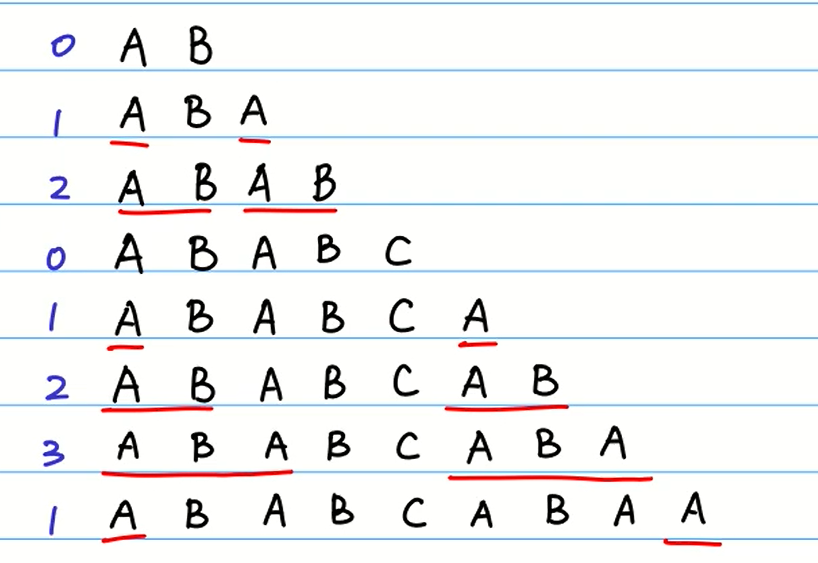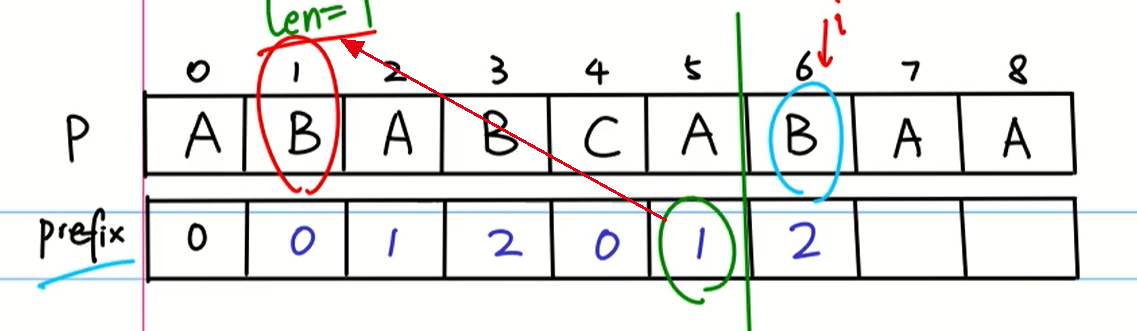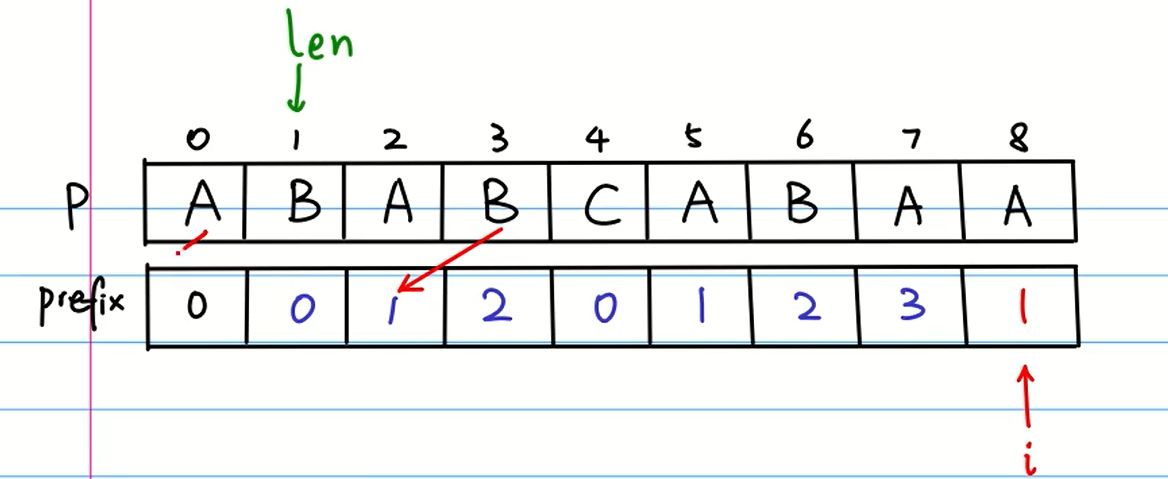kmp算法原理和实现，转自：从头到尾彻底理解KMP（2014年8月22日版）

# 暴力算法

• 如果当前字符串串匹配成功，即$S[i]==p[j]$，则$i++,j++$，继续匹配下一个字符；
• 如果失败，即$S[i] != P[j]$，则令i=i-(j-1),j=0，相等于每次匹配失败时，$i$回溯，$j$被重置为0。

1. S为B，P为A，不匹配，执行第②条指令：“如果失配（即S[i]! = P[j]），令i = i - (j - 1)，j = 0”，S跟P匹配，相当于模式串要往右移动一位（i=1，j=0）2. S跟P还是不匹配，继续执行第②条指令：“如果失配（即S[i]! = P[j]），令i = i - (j - 1)，j = 0”，S跟P匹配（i=2，j=0），从而模式串不断的向右移动一位（不断的执行“令i = i - (j - 1)，j = 0”，i从2变到4，j一直为0）3. 直到S跟P匹配成功（i=4，j=0），此时按照上面的暴力匹配算法的思路，转而执行第①条指令：“如果当前字符匹配成功（即S[i] == P[j]），则i++，j++”，可得S[i]为S，P[j]为P，即接下来S跟P匹配（i=5，j=1）4. S跟P匹配成功，继续执行第①条指令：“如果当前字符匹配成功（即S[i] == P[j]），则i++，j++”，得到S跟P匹配（i=6，j=2），如此进行下去5. 直到S为空格字符，P为字符D（i=10，j=6），因为不匹配，重新执行第②条指令：“如果失配（即S[i]! = P[j]），令i = i - (j - 1)，j = 0”，相当于S跟P匹配（i=5，j=0）6. 至此，我们可以看到，如果按照暴力匹配算法的思路，尽管之前文本串和模式串已经分别匹配到了S、P，但因为S跟P不匹配，所以文本串回溯到S，模式串回溯到P，从而让S跟P匹配。# KMP算法

KMP主要分为三个步骤：

• 构造前缀表，即prefix table
• 前缀表后移，并将第一个元素置为-1
• KMP查找主体KMP算法实现：